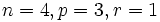# Projective special linear group equals alternating group in only finitely many cases

## Statement

The following are the cases where a Projective special linear group (?) is isomorphic to an Alternating group (?):

1.$PSL(1,k)$ is isomorphic to the alternating group on one letter, and on two letters, for any field$k$.
2.$PSL(2,3)$ is isomorphic to the alternating group of degree four.
3.$PSL(2,4)$ and$PSL(2,5)$ are both isomorphic to the alternating group of degree five.
4.$PSL(2,9)$ is isomorphic to the alternating group of degree six.
5.$PSL(4,2)$ is isomorphic to the alternating group of degree eight.

## Proof

### The case of degree more than two

We first consider the case where$m \ge 3$. In this case, we prove that the only solution is$m = 4, q = 2, n = 8$.

### The case of characteristic two

We now consider the case of fields of characteristic two. In this case, the group is:$\! PSL(2,2^r)$

Its order is given by:$\! (2^r + 1)2^r (2^r - 1)$.

For this to be isomorphic to the alternating group$A_n$, we must have:$\! (2^r + 1)2^{r+1}(2^r - 1) = n!$.

Note that both$2^r + 1$ and$2^r - 1$ are both odd, so the largest power of$2$ dividing$n!$ is$r + 1$. This yields:$\! r + 1 \le n - 1$.

Thus,$\! r \le n - 2$. We thus have:$\! n! = 2^{r + 1} (2^{2r} - 1) \le 2^{n-1}(2^{2n - 4} - 1) \le 2^{3n - 5}$.

This puts a small bound on$n$, namely,$n \le 14$.as well as on$r$, namely$r \le 12$. A hand calculation shows that the only solutions are$r = 2, n = 5$ and$r = 3,n = 8$. A further check shows that in this case, the groups are indeed isomorphic.

### The case of odd characteristic

We now consider the case of an odd prime, so the group is:$\! PSL(2,p^r)$.

The order of the group is:$\! p^r(p^r + 1)(p^r - 1)/2$.

For this to be isomorphic to the alternating group$A_n$, we get:$\! p^r(p^r + 1)(p^r - 1) = n!$.

Clearly,$p^r + 1$ and$p^r - 1$ are relatively prime to$p$. Thus, the largest power of$p$ dividing$n!$ is$p^r$. This yields:$r \le \frac{n}{p-1}$.

Thus, we get:$n! \le p^{3n/(p-1)}$.

Note that since$\! p^{3/(p-1)} < 8$ for all primes$p$, we get:$\! n! < 8^n$.

This puts a bound$n \le 19$. A hand calculation now yields that we have the following solutions:

•$\! n = 4, p = 3, r = 1$.
•$\! n = 5, p = 5, r = 1$.
•$\! n = 6, p = 3, r = 2$.